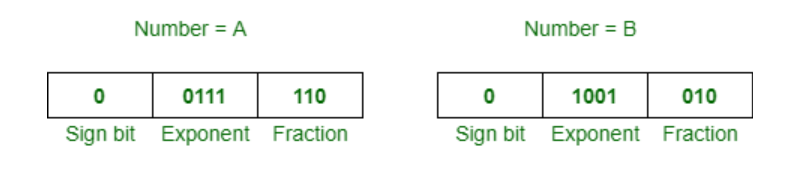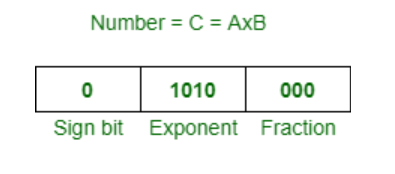# Multiplying Floating Point Numbers

• Last Updated : 04 May, 2020

Prerequisite – IEEE Standard 754 Floating Point Numbers
Problem:-
Here, we have discussed an algorithm to multiply two floating point numbers, x and y.

Algorithm:-

1. Convert these numbers in scientific notation, so that we can explicitly represent hidden 1.
2. Let ‘a’ be the exponent of x and ‘b’ be the exponent of y.
3. Assume resulting exponent c = a+b. It can be adjusted after the next step.
4. Multiply mantissa of x to mantissa of y. Call this result m.
5. If m does not have a single 1 left of radix point, then adjust radix point so it does, and adjust exponent c to compensate.
6. Add sign bits, mod 2, to get sign of resulting multiplication.
7. Convert back to one byte floating point representation, truncating bits if needed.

Note :
Negative values are simple to take care of in floating point multiplication. Treat sign bit as 1 bit unsigned binary, add mod 2. This is the same as XORing the sign bit.

Example :-
Suppose you want to multiply following two numbers:Now, these are steps according to above algorithm:

1. Given, A = 1.11 x 2^0 and B = 1.01 x 2^2
2. So, exponent c = a + b = 0 + 2 = 2 is the resulting exponent.
3. Now, multiply 1.11 by 1.01, so result will be 10.0011
4. We need to normalize 10.0011 to 1.00011 and adjust exponent 1 by 3 appropriately.
5. Resulting sign bit 0 (XOR) 0 = 0, means positive.
6. Now, truncate and normalite it 1.00011 x 2^3 to 1.000 x 2^3.

Therefore, resultant number is,Similarly, we can multiply other floating point numbers.

My Personal Notes arrow_drop_up